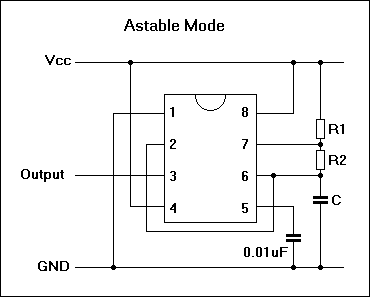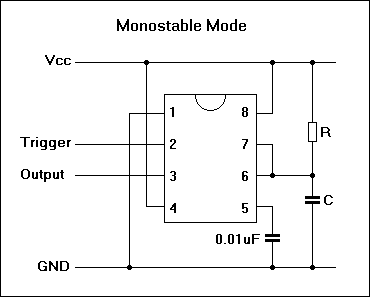## IC 555 Timer (monostable and astable modes) CalculatorR1:
R2:
C:

T1 Output High (Seconds) =
T2 Output Low (Seconds) =
T Total Period (Seconds) =
Frequency (Hz) =
Duty (%High) =

T1 = 0.7(R1 + R2)C and T2 = 0.7 R1 C

So T = 0.7 (R1 + 2R2)C or f = 1.45/((R1 + 2R2)C)

Where T1 = High Period, T2 = Low Period,T = Total Period, f = Frequency.R:
C:

T Output High (Seconds) =

The 555 timer Calculator to get 555 chip in both monostable and astable modes.

Thinkcalculator.com provides you helpful and handy calculator resources.